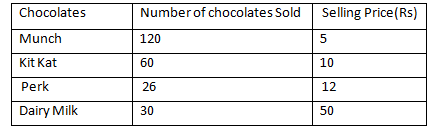# SSC CGL 2018 Practice Test Papers | Quantitative Aptitude (Day-25)

Dear Aspirants, Here we have given the Important SSC CGL Exam 2018 Practice Test Papers. Candidates those who are preparing for SSC CGL 2018 can practice these questions to get more confidence to Crack SSC CGL 2018 Examination.

[WpProQuiz 3049]

Click “Start Quiz” to attend these Questions and view Explanation

Directions (1- 3): The table gives sales of different Chocolates in a shop.1) Find the average of selling price of Munch and Kitkat.

a) 5

b) 120

c) 7.5

d) 12

2) If the seller gets 25% profit by selling Dairy milk. Find the cost price of 25 dairy milk.

a) 700

b) 1000

c) 2000

d) 1250

3) Find the difference between number of Perk and Diary Milk chocolates sold?

a) 4

b) 10

c) 20

d) 38

4) What is the digit in tens place in the product of first 20 natural numbers?

a) 3

b) 2

c) 0

d) 5

5) The LCM and HCF of two numbers are 360 and 18 respectively. How many such pairs are possible?

a) 3

b) 2

c) 5

d) 1

6) There are three numbers A, B and C, Six times of A is equals to 7 times of B. Five times of B is equals to 6 times of C. If A is 20 more than C. Find the third number C.

a) 10

b) 25

c) 20

d) 40

7) The ratio of two numbers is 3/4:7/8. By what percentage is the first number more/less than the second number?

a) 19.19% more

b) 14.28 % more

c) 20 % less

d) 25% less

8) The average weight of a class is 40 kg. Which of the following can be concluded?

a) If three students whose weights are 49 kg, 37 kg and 36 kg join the class, the average weight of the class will decrease.

b) ) If three students whose weights are 49 kg, 37 kg and 36 kg leave the class, the average weight of the class will increase

c) Neither a or b

d) Both and b

9) In a 200 m race, A beats B by 20 m or 10 seconds. What distance should be the head start that A can give B in the same race, so that A and B will finish the race at same time?

a) 30 m

b) 10 m

c) 20 m

d) 25 m

10) Simplify: 35+ 20/100 x 50% of 230

a) 56

b) 60

c) 58

d) 55

Selling price of Munch =5

Selling price of Kit Kat = 10

Sum of SP of Munch and Kitkat =10+5=15

Average= 15/2=7.5

SP of Dairy milk= 50

Profit=25%

CP=50/125×100=40

CP of 25 diary milk =40×25=1000

30 – 26 = 4

The numbers from 1 to 20 have numbers 10, 20.

10 x 20 = 200

Product of these two will obviously have 0 in tens place. So, product of first 20 natural numbers will also have 0 in tens place.

LCM x HCF = Product of two numbers.

Let the two numbers be 18a and 18b respectively, where a and b are coprimes.

18×360 =324 ab

=> ab= 20

20 can be expressed as a product of two factors in 2 ways: (1×20) and (4×5)

Both pairs are co-prime. So the set of possible pairs are (18, 360) and (72, 90)

6A=7B=> A:B= 7:6

5B=6C=> B:C=6:5

A:B:C= 7:6:5

7x-5x=20

2x=20=>x=10

C=5x=50

¾:7/8= 24:28= 6:7

Percentage difference= 1/7×100=14.28%

Average of 49,37and 36=122/3=40.66

It is more than the average 40, so if three students whose weights are 49 kg, 37 kg and 36 kg join the class, the average weight of the class will increase.

Similarly, if three students whose weights are 49 kg, 37 kg and 36 kg leave the class, the average weight of the class will decrease.

Distance covered by A when A finish the Race=180 m

B covers 20 m in 10 seconds.

Speed of B= 20/10= 2 m/s

To finish in same time, B should start 10 seconds before A.

Distance covered by B in 10 seconds= 10×2=20 m

So A should give B a head start of 20 m.